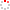#CodeStepByStep## switch_pairs

Language/Type: PHP arrays collections

Write a function named `switch_pairs` that takes an array as a parameter and returns a new array with the contents of the passed array switched in a pairwise fashion. That is, the order of the first two values in the passed array should be switched in the result array, then the order of the next two, then the order of the next two, and so on.

For example, if the array passed stores `[1, 4, 8, -3, 2, 7]`, then the returned array should switch the first pair, (1 and 4), the second pair (8 and -3) and the third pair (2 and 7) to yield `[4, 1, -3, 8, 7, 2]`.

If there are an odd number of values in the array, the final element is not moved. For example, if the original array had been `[1, 2, 3, 4, 5]`, then the result returned would be `[2, 1, 4, 3, 5]`.

Constraints:

• Do not modify the passed array.
Type your PHP solution code here:

This is a function exercise. Write a PHP function as described. Do not write a complete program; just the function(s) above.